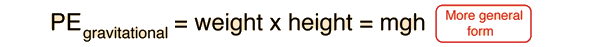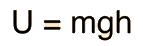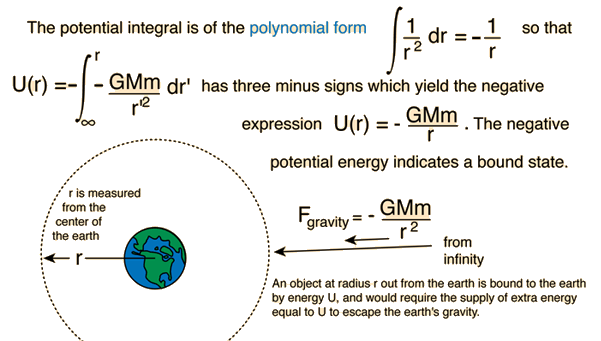# Gravitational Potential Energy

Gravitational potential energy is energy an object possesses because of its position in a gravitational field. The most common use of gravitational potential energy is for an object near the surface of the Earth where the gravitational acceleration can be assumed to be constant at about 9.8 m/s2. Since the zero of gravitational potential energy can be chosen at any point (like the choice of the zero of a coordinate system), the potential energy at a height h above that point is equal to the work which would be required to lift the object to that height with no net change in kinetic energy. Since the force required to lift it is equal to its weight, it follows that the gravitational potential energy is equal to its weight times the height to which it is lifted.PE = kg x 9.8 m/s2 xm = joules.
PE = lbs xft = ft lb.

Index

Energy concepts

 HyperPhysics***** Mechanics R Nave
Go Back

# Gravitational Potential Energy

The general expression for gravitational potential energy arises from the law of gravity and is equal to the work done against gravity to bring a mass to a given point in space. Because of the inverse square nature of the gravity force, the force approaches zero for large distances, and it makes sense to choose the zero of gravitational potential energy at an infinite distance away. The gravitational potential energy near a planet is then negative, since gravity does positive work as the mass approaches. This negative potential is indicative of a "bound state"; once a mass is near a large body, it is trapped until something can provide enough energy to allow it to escape. The general form of the gravitational potential energy of mass m is:where G is the gravitation constant, M is the mass of the attracting body, and r is the distance between their centers.

This is the form for the gravitational potential energy which is most useful for calculating the escape velocity from the earth's gravity.

Index

Energy concepts

 HyperPhysics***** Mechanics R Nave
Go Back

# Gravitational Potential Energy

From the work done against the gravity force in bringing a mass in from infinity where the potential energy is assigned the value zero, the expression for gravitational potential energy isThis expression is useful for the calculation of escape velocity, energy to remove from orbit, etc. However, for objects near the earth the acceleration of gravity g can be considered to be approximately constant and the expression for potential energy relative to the Earth's surface becomeswhere h is the height above the surface and g is the surface value of the acceleration of gravity.

Index

Energy concepts

Gravity concepts

 HyperPhysics***** Mechanics R Nave
Go Back

# Gravitational PotentialIndex

Energy concepts

 HyperPhysics***** Mechanics R Nave
Go Back

# Gravitational Potential IntegralIndex

Energy concepts

 HyperPhysics***** Mechanics R Nave
Go Back